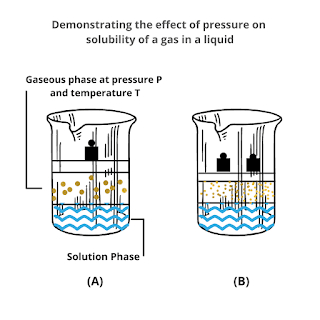## Posts

Showing posts from April, 2021

### Henry's Law: Definition, Formula, Applications and LimitationsWhat is Henry's Law? Henry's law deals with the solubility of the gas in a liquid at a particular temperature. This law was given by Henry in 1803 which states that The mass of a gas dissolved in a given volume of the liquid at constant temperature is directly proportional to the pressure of the gas present in equilibrium with the liquid. We can understand Henry's law by a simple experiment. Let us consider a dynamic equilibrium system shown in the given figure. The lower part of the system is liquid and its upper part is filled with a gas having pressure P and temperature T. Now, slowly increase the pressure over the system as shown in the figure. You will notice that the concentration of particles of gas in the liquid increases i.e gas is getting dissolved in the liquid. The more you increase the pressure, the more gas will dissolve in the liquid till a saturation point is attained. This is nothing but Henry's law. Mathematical Derivation of Henry's Law Mathema